# Chemistry Facts for Kids

Welcome to our Chemistry Facts library. On this page you can access one of the largest collections of facts about the chemical elements on the periodic table. This is an excellent resource for students, teachers or anyone who wants to learn more about an element on the periodic table. We hope you find these facts about the elements informative and educational. You can begin to explore our chemistry facts library by scrolling down and choosing a section.

Our Chemistry Facts category is separated into different sections. Each of the below sections is about a specific chemical element. For example, we have a section about Hydrogen, Carbon and Oxygen. We're constantly adding new elements, if you can't find facts for a specific element then you should check back often. You can also contact us with your requests, and we'll add it to one of our future updates.

Each of the available chemistry facts sections is listed below, in order of their atomic number.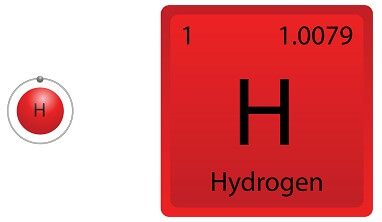## Hydrogen Facts

In this section you'll learn about hydrogen. You'll learn what its symbol is, the atomic number for hydrogen, the atomic weight of hydrogen and other hydrogen facts.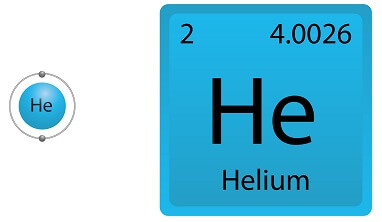## Helium Facts

In this section you'll learn about helium. You'll learn what its symbol is, the atomic number for helium, the atomic weight of helium and other helium facts.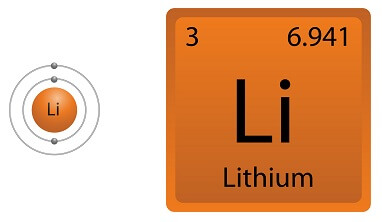## Lithium Facts

In this section you'll learn about lithium. You'll learn what its symbol is, the atomic number for lithium, the atomic weight of lithium and other lithium facts.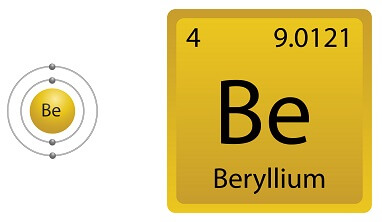## Beryllium Facts

In this section you'll learn about beryllium. You'll learn what beryllium's symbol is, the atomic number for beryllium, it's atomic weight and other beryllium facts.## Boron Facts

In this section you'll learn about boron. You'll learn what boron's symbol is, the atomic number for boron, the atomic weight of boron and other boron facts.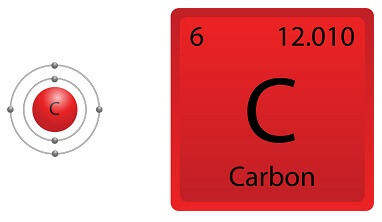## Carbon Facts

In this section you'll learn about carbon. You'll learn what its symbol is, the atomic number for carbon, the atomic weight of carbon and other carbon facts.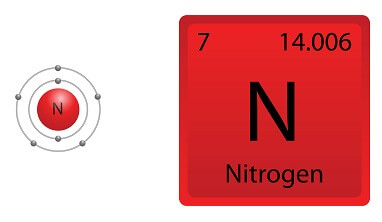## Nitrogen Facts

In this section you'll learn about nitrogen. You'll learn what its symbol is, the atomic number for nitrogen, the atomic weight of nitrogen and other nitrogen facts.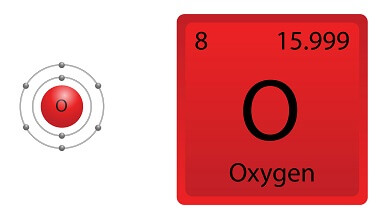## Oxygen Facts

In this section you'll learn about oxygen. You'll learn what its symbol is, the atomic number for oxygen, the atomic weight of oxygen and other oxygen facts.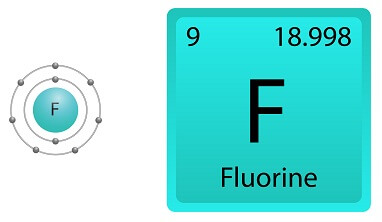## Fluorine Facts

In this section you'll learn about fluorine. You'll learn what its symbol is, the atomic number for fluorine, the atomic weight of fluorine and other fluorine facts.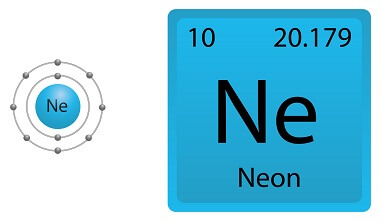## Neon Facts

In this section you'll learn about neon. You'll learn what its symbol is, the atomic number for neon, the atomic weight of neon and other neon facts.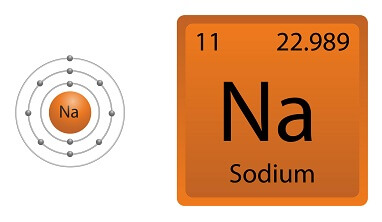## Sodium Facts

In this section you'll learn about sodium. You'll learn what its symbol is, the atomic number for sodium, the atomic weight of sodium and other sodium facts.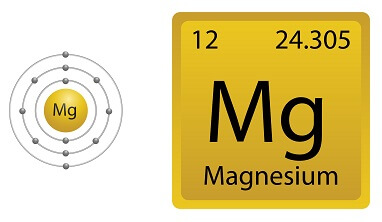## Magnesium Facts

In this section you'll learn about magnesium. You'll learn what its symbol is, the atomic number for magnesium, its atomic weight and other magnesium facts.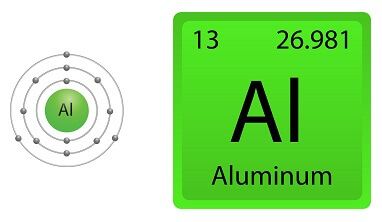## Aluminium Facts

In this section you'll learn about aluminium. You'll learn what its symbol is, the atomic number for aluminium, the atomic weight of aluminium and other aluminium facts.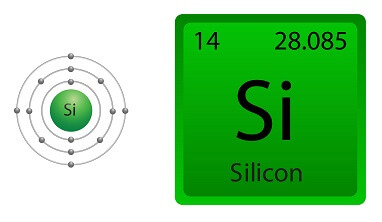## Silicon Facts

In this section you'll learn about silicon. You'll learn what its symbol is, the atomic number for silicon, the atomic weight of silicon and other silicon facts.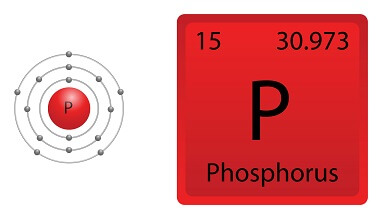## Phosphorus Facts

In this section you'll learn about phosphorus. You'll learn what its symbol is, the atomic number for phosphorus, its atomic weight and other phosphorus facts.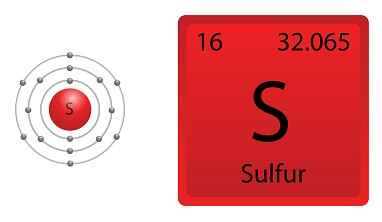## Sulfur Facts

In this section you'll learn about sulfer. You'll learn what its symbol is, the atomic number for sulfer, the atomic weight of sulfer and other sulfer facts.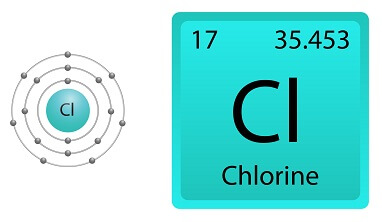## Chlorine Facts

In this section you'll learn about chlorine. You'll learn what its symbol is, the atomic number for chlorine, the atomic weight of chlorine and other chlorine facts.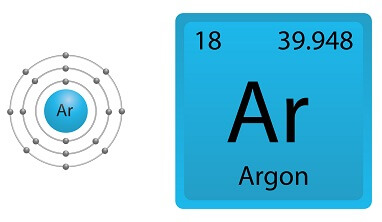## Argon Facts

In this section you'll learn about argon. You'll learn what argon's symbol is, the atomic number for argon, the atomic weight of argon and other argon facts.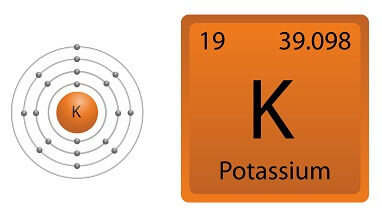## Potassium Facts

In this section you'll learn about potassium. You'll learn what its symbol is, the atomic number for potassium, the atomic weight of potassium and other potassium facts.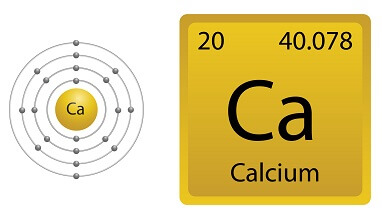## Calcium Facts

In this section you'll learn about calcium. You'll learn what its symbol is, the atomic number for calcium, the atomic weight of calcium and other calcium facts.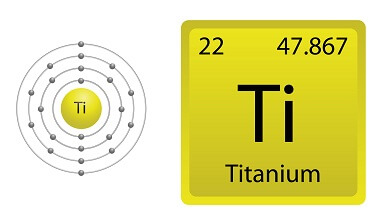## Titanium Facts

In this section you'll learn about titanium. You'll learn what its symbol is, the atomic number for titanium, the atomic weight of titanium and other titanium facts.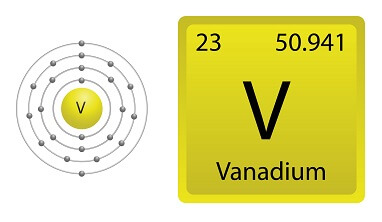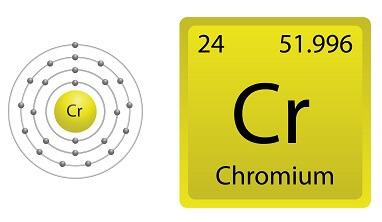## Chromium Facts

In this section you'll learn about chromium. You'll learn what its symbol is, the atomic number for chromium, the atomic weight of chromium and other chromium facts.## Manganese Facts

In this section you'll learn about manganese. You'll learn what its symbol is, the atomic number for manganese, its atomic weight and other manganese facts.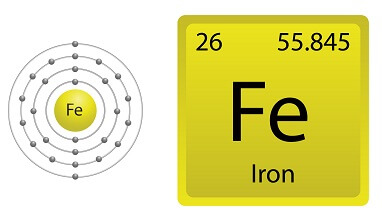## Iron Facts

In this section you'll learn about iron. You'll learn what its symbol is, the atomic number for iron, the atomic weight of iron and other iron facts.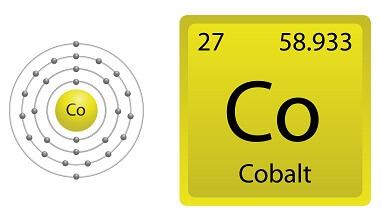## Cobalt Facts

In this section you'll learn about cobalt. You'll learn what its symbol is, the atomic number for cobalt, the atomic weight of cobalt and other cobalt facts.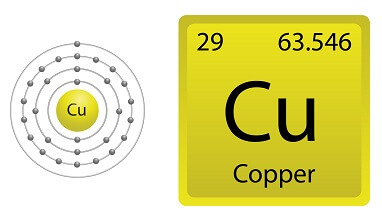## Copper Facts

In this section you'll learn about copper. You'll learn what its symbol is, the atomic number for copper, the atomic weight of copper and other copper facts.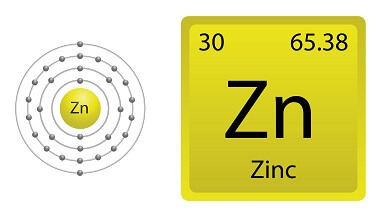## Zinc Facts

In this section you'll learn about zinc. You'll learn what its symbol is, the atomic number for zinc, the atomic weight of zinc and other facts about zinc.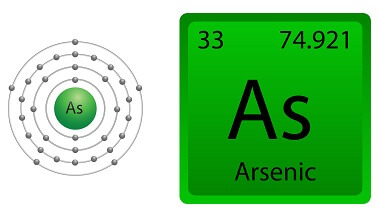## Arsenic Facts

In this section you'll learn about arsenic. You'll learn what arsenic's symbol is, the atomic number for arsenic, the atomic weight of arsenic and other arsenic facts.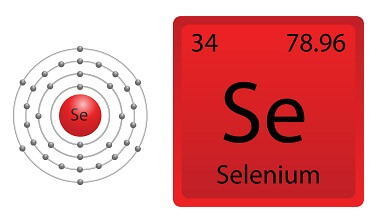## Selenium Facts

In this section you'll learn about selenium. You'll learn what its symbol is, the atomic number for selenium, the atomic weight of selenium and other selenium facts.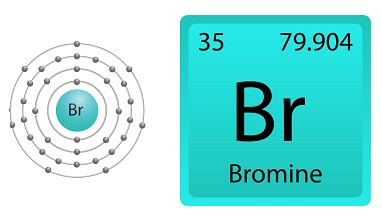## Bromine Facts

In this section you'll learn about bromine. You'll learn what bromine's symbol is, the atomic number for bromine, the atomic weight of bromine and other bromine facts.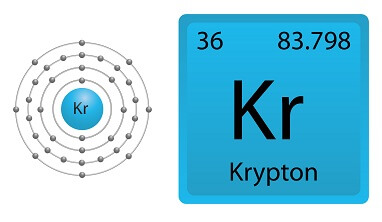## Krypton Facts

In this section you'll learn about krypton. You'll learn what krypton's symbol is, the atomic number for krypton, the atomic weight of krypton and other krypton facts.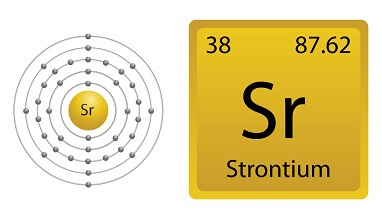## Strontium Facts

In this section you'll learn about strontium. You'll learn what its symbol is, the atomic number for strontium, the atomic weight of strontium and other strontium facts.## Silver Facts

In this section you'll learn about silver. You'll learn what its symbol is, the atomic number for silver, the atomic weight of silver and other silver facts.## Tin Facts

In this section you'll learn about tin. You'll learn what its symbol is, the atomic number for tin, the atomic weight of tin and other tin facts.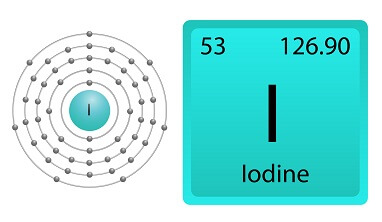## Iodine Facts

In this section you'll learn about iodine. You'll learn what its symbol is, the atomic number for iodine, the atomic weight of iodine and other iodine facts.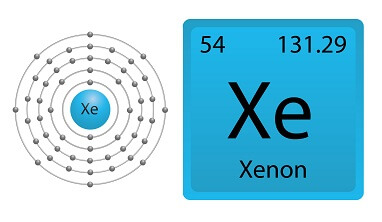## Xenon Facts

In this section you'll learn about xenon. You'll learn what its symbol is, the atomic number for xenon, the atomic weight of xenon and other xenon facts.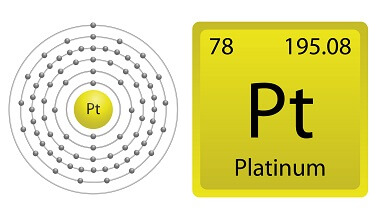## Platinum Facts

In this section you'll learn about platinum. You'll learn what its symbol is, the atomic number for platinum, the atomic weight of platinum and other platinum facts.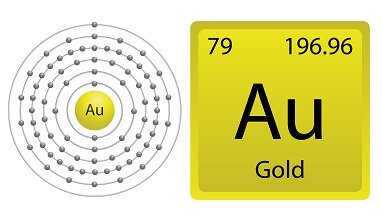## Gold Facts

In this section you'll learn about gold. You'll learn what its symbol is, the atomic number for gold, the atomic weight of gold and other gold facts.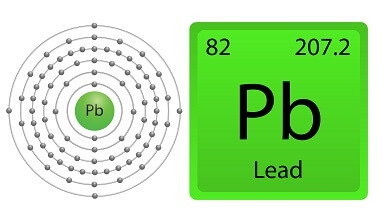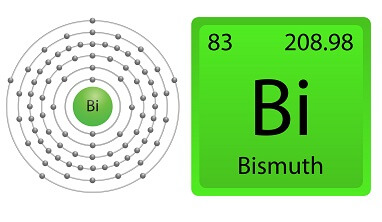## Bismuth Facts

In this section you'll learn about bismuth. You'll learn what bismuth's symbol is, the atomic number for bismuth, the atomic weight of bismuth and other bismuth facts.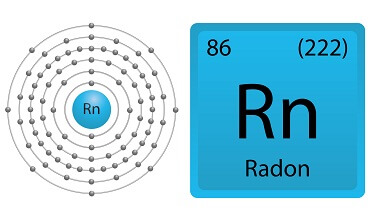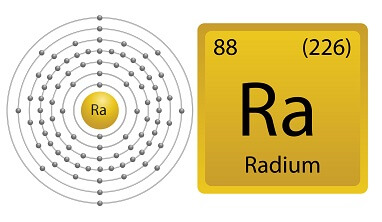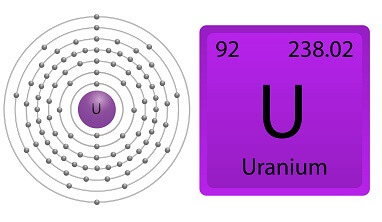## Uranium Facts

In this section you'll learn about uranium. You'll learn what its symbol is, the atomic number for uranium, the atomic weight of uranium and other uranium facts.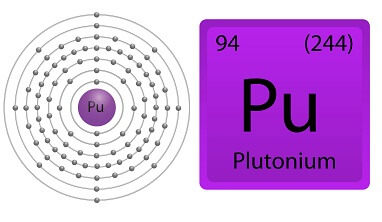## Plutonium Facts

In this section you'll learn about plutonium. You'll learn what its symbol is, the atomic number for plutonium, the atomic weight of plutonium and other plutonium facts.

The periodic table of elements (periodic table) is a table that contains all the known chemical elements. The periodic table today contains 94 chemical elements that are natural and 24 that have only been synthesized in a lab, in a nuclear reactor, or in a nuclear explosion. Each chemical element on the periodic table is assigned an element name, an atomic number and its atomic weight. The atomic number is how many protons are in the nucleus of every atom of that element. The atomic weight is the total number of protons and neutrons in the atom.

The 94 naturally occurring elements on the periodic table belong to one of seven periods and one of four blocks. Periods are horizontal rows on the periodic table, so if a chemical element is on row three it's in period three. The blocks on the periodic table go from left to right in the following order s-block, f-block, d-block and b-block. The blocks on the periodic table represent the sequence in which the electron shells of the elements are filled.

The 94 naturally occurring elements on the periodic table belong to one of eighteen groups. The eighteen groups on the periodic table are the lithium group, beryllium group, scandium group, titanium group, vanadium group, chromium group, manganese group, iron group, cobalt group, nickel group, copper group, zinc group, boron group, carbon group, nitrogen group, oxygen group, fluorine group and helium group.